# Interior Lighting Design Methods- Part Two

I indicted before in our course, that we can design interior lighting by using any method from the following three ones:

1. The Zonal Cavity (Lumen) method
2. Point by point method
3. Watt per square feet method

and in the previous article " Interior Lighting Design Methods- Part One ", I explained the first (8) steps in Zonal cavity (Lumen) method calculations by using IES Procedures

Today I will explain other steps in
Zonal cavity (Lumen) method calculations by using IES Procedures.

You can review the following previous articles for more information and good following:

Step#9: calculate the number of required luminaires

No. of luminaires = (Awp x Ewp )/ ((lumens/lamp) x (lamps/luminaires) x CUXLLF)

Where:

Ewp = average maintained illuminance on the work plane

Φ (TOTAL) = total system lamp lumen output

CU = coefficient of utilization

LLF TOTAL = Total light loss factor

Awp = area of the work plane

Example #6:

Find the number of luminaires needed in an office given the following:

• office dimensions: 9.15m by 9.15m by 3.5m
• Working Plane Height: 0.76m
• Reflectances (ρ): Ceiling cavity 0.70
• Walls 0.30
• Floor Cavity 0.20
• Assume LLF TOTAL = 0.75

Step#1: By using the following table, Determine the “Type of Activity” to determine its “Illuminance Category”

given that Type of activity = office

so, Illuminance Category = D

Step#2: determine the “Range of Illuminance” from the chart of IESNA illuminance Recommendations  (in above table) or other standards.

for activity office and
Illuminance Category D :

Range of Illuminance = 200 /300/500 LUX

Step#3: Using Table b in below, determine the weighting factors applicable to the illuminance category D.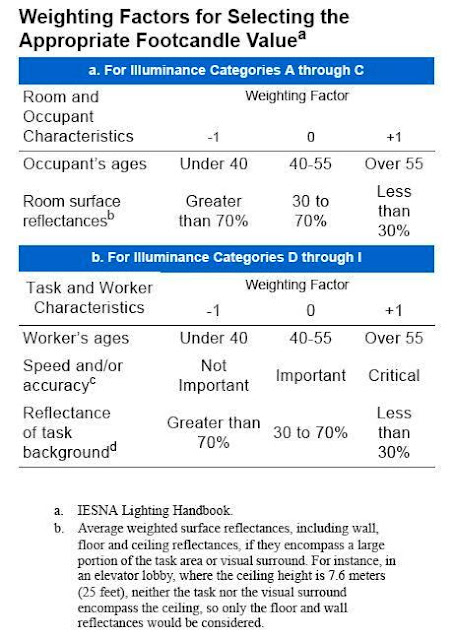Worker’s age = 40-55 gives weighting factor= 0

Speed and /or accuracy is critical gives weighting factor= +1

Reflectance of task background less than 30% gives weighting factor= +1

Sum of weighting factors = +2

So, the middle illuminance value = 300 Lux will be selected.

Step#4: select a suitable lighting luminarie from the manufacturer’s catalogs for the activity and place under design.

We select Luminaire: Recessed round

Lamp: 70 watt metal halide, 5600 lumen initial output

Step#5: Calculations of Room, Ceiling and Floor Cavity Ratios (see fig.1)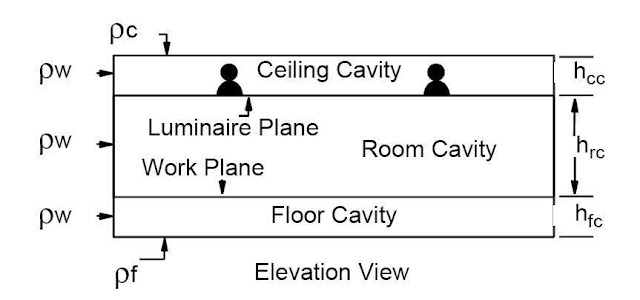Fig.1

W = Room Width= L = Room Length= 9.15 m

hcc = height of ceiling cavity. For recessed fixtures hcc = 0

hfc = height of floor cavity = 0.76

hrc = height of room cavity = 3.49-0.76 = 2.73

RCR for square room
will be calculated as follows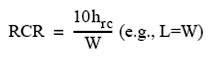so, RCR = 10 x2.73 / 9.15 = 2.98 = 3

And, FCR will be calculated as follows

so, FCR =10 X 0.76 / 9.15 = 0.83

Step#6: Determination of Room surface reflectances

Given in input data that :

Effective Ceiling Cavity Reflectance (ρCC) = 0.70

Walls Cavity Reflectance (rw )= 0.30

Note: you usually do estimation for these values based on the typical approximate Reflectances indicated in the below table: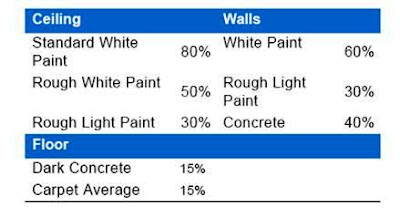Step#7: Determination of Coefficient of Utilization (CU)

By using ρCC and rw obtained from step# 6 and by using Tables in CU determination for metal halide lamps in below.

so, We get that CU = 0.55

Step#8: Calculate Light Loss Factor (LLF)

Given in input data that :

LLF TOTAL = 0.75

Note: you can use the criteria developed by IESNA for getting an approximate value for the LLF TOTAL ,you can download this Criteria by clicking on the link.

Step#9: calculate the number of required luminaires

Number of luminaires = (AWP x EWP ) / (lumens/lamp x lamps/luminaires x CU x LLF TOTAL)

Where:

Ewp = average maintained illuminance on the work plane

Φ (TOTAL) = total system lamp lumen output

CU = coefficient of utilization

LLF TOTAL = Total light loss factor

Awp = area of the work plane

Number of luminaires =( 9.15m by 9.15m x 300 lux )/ (5600 lumen x 1 x 0.55 x 0.75) = 10.9

In this example, 12 fixtures can be spaced uniformly in a 3 by 4 pattern.

Although 12 is more than the calculated value of 10.9 fixtures, results within a 10% margin is generally acceptable for meeting this target criterion

Step#10: Calculate of Total System Lamp Lumen Output Φ (TOTAL):

Φ (TOTAL) = (nos. of luminaires) X (number of lamps/ luminaire) X (Lumen of one lamp)

Example #7:

In an office space 3m x 4.6m with a 2.6m ceiling height, there are 2 recessed fluorescent luminaires. Each luminaire has three (3) 32W 48” T8 fluorescent lamps. Manufacturer’s data shows that the initial lumen output of the lamp is 2900 lumens. What is the total lamp lumen output Φ (TOTAL)?

Φ(TOTAL) = 2 luminaires x 3 lamps/luminaire x 2900 lumens/lamp = 17,400 lumens

Step#11: calculate the illuminance on the workplane by using the following equation: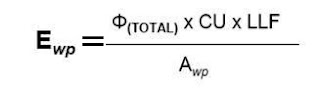Where:

Ewp = average maintained illuminance on the work plane

Φ (TOTAL) = total system lamp lumen output

CU = coefficient of utilization

LLF = light loss factor

Awp = area of the work plane

Example#8:

Substituting all the computed values in Examples # 2, 4, 5 in the previous article & example#7 in above and using the equation for average illuminance on the workplane, we have:

EWP = (17,400 lm x 0.50 x 0.75)/(3.05m x 4.57m) = 468 lm/m2 or 486 lux (Maintained)
The average initial illuminance on the workplane can be determined by substituting only the non-recoverable light loss factors for the total light loss factor.

EWP = (17,400 lm x 0.50 x 0.0.93)/ (3.05m x 4.57m) = 581 lm/m2 or 581 lux (Initial)

Notes for example# 8:

• An average maintained illuminance of 468 lumens per square meter will strike the area covered by the workplane in a completely empty space.
• Some points on the workplane will have an illuminance higher than 468 while others will have an illuminance lower than this value.
• During first time that this system will be turned on, wherein the lamps are new and the surfaces are clean, the average initial illuminance will be greater than the maintained value, which is computed as 582 lumens per square meter (lux).

The spacing criteria

• Spacing Criteria is the maximum ratio of spacing to mounting height of the luminaire above the workplane that provides reasonable uniformity of illumination within the space.
• i.e , S/H (space to height ratio) = space between luminaires / height of the luminaire above the workplane.
• So we have two S/H, one in X direction (Rows or Axial direction) and one in Y direction (Columns or Transverse direction).
• S/H ratio, usually between 0.5 to 1.5,
• S/H ratio X the mounting height = the maximum distance that the luminaires maybe separated and provide uniform illuminance on the workplane.
• Spacing ratios for specific luminaires are given in the data sheets published by each manufacturer (see fig.2).Fig.2

A- For fluorescent luminaires:• The maximum number of luminaires that can be installed in one row = (the room length - at least 0.3 meter) / the length of the luminaire.
• The exact spacing between rows = the room width / the number of rows.
• Spacing between luminaires in each row = the room length / the number of luminaires per row.
• Spacing between the outer luminaires and the adjacent wall = onehalf of the luminaire spacing.
• If desks or other work areas are to be located alongside the walls, then the wall-to-luminaires spacing = one-third of the luminaire spacing.

B- For luminaires using essentially point sources of light, such as incandescent or HID lamps:The number of luminaires per row should be in proportion to the width-to-length ratio of the room.

Note:
the best S/H ratio is between 1 and 1.25 which verify uniform illuminance on the workplane and economic solution for selected quantity of luminaires.

In the next article, I will explain the point by point method for lighting design. Please, keep following.

#### 1 comment:

1.Dear brother Ali,
Can you please provide the details of lamps with their standard names like T5, T8, T2 etc. What does this standard names signify and share the pdf for that if u have.

Regards